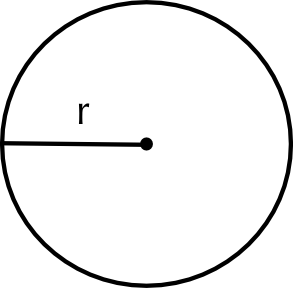Home Practice
For learners and parents For teachers and schools
Textbooks
Full catalogue
Pricing SupportLog in

We think you are located in United States. Is this correct?

# 9.3 Area

## 9.3 Area (EMG5V)

Area
The size of a surface or plane, or the amount of space inside the boundary of a flat ($$\text{2}$$ dimensional) object such as a square, triangle or circle. area is measured in mm$$^{\text{2}}$$ ; cm$$^{\text{2}}$$ ; m$$^{\text{2}}$$ or km$$^{\text{2}}$$.

### Estimating area (EMG5W)

We can estimate area without using any formulae. One way of doing this is to place a square grid over the area we wish to estimate. If we know the size of the squares, we can then count up how many squares are filled by the area we wish to measure. As we shall see, for some shapes, this method is easy to use and accurate (we would get the same answer for our area as we would using a formula). For other shapes it becomes harder to estimate area accurately however, because they don't fit neatly into a square grid.

## Worked example 4: Estimating the area of a rectangle using a grid

Using the grid below, estimate the area (in cm$$^{\text{2}}$$) of Mr and Mrs Dlamini's rectangular patio. Each square in the grid is $$\text{1}$$ $$\text{cm}$$ by $$\text{1}$$ $$\text{cm}$$.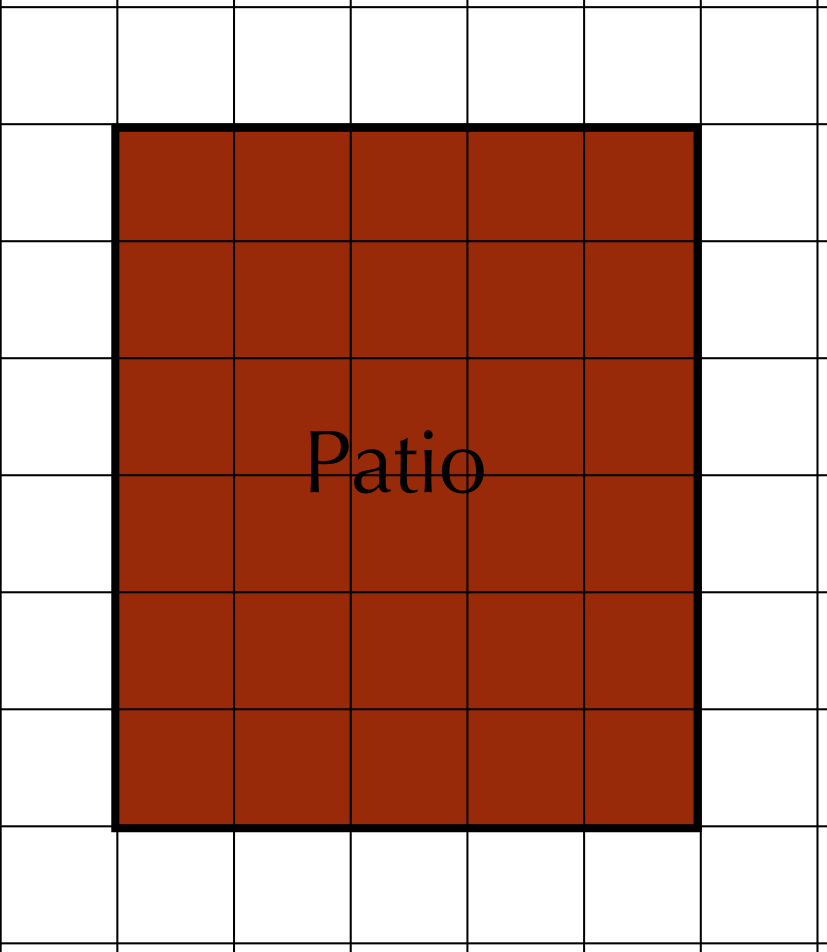Count the number of blocks and make a mark with your pencil in each one as you count it. This will help you check that you have counted every block.

The patio is $$\text{5}$$ blocks wide and $$\text{6}$$ blocks high. $$\text{6}$$ rows of $$\text{5}$$ blocks = $$\text{6}$$ $$\times$$ $$\text{5}$$ = $$\text{30}$$ blocks.

Each block is $$\text{1}$$ $$\text{cm}$$$$^{\text{2}}$$ so $$\text{30}$$ blocks = $$\text{30}$$ $$\text{cm}$$$$^{\text{2}}$$.

## Worked example 5: Estimating the area of a triangle using a grid

Using the grid below, estimate the area of the triangular garden. Each square in the grid is $$\text{1}$$ $$\text{cm}$$ by $$\text{1}$$ $$\text{cm}$$.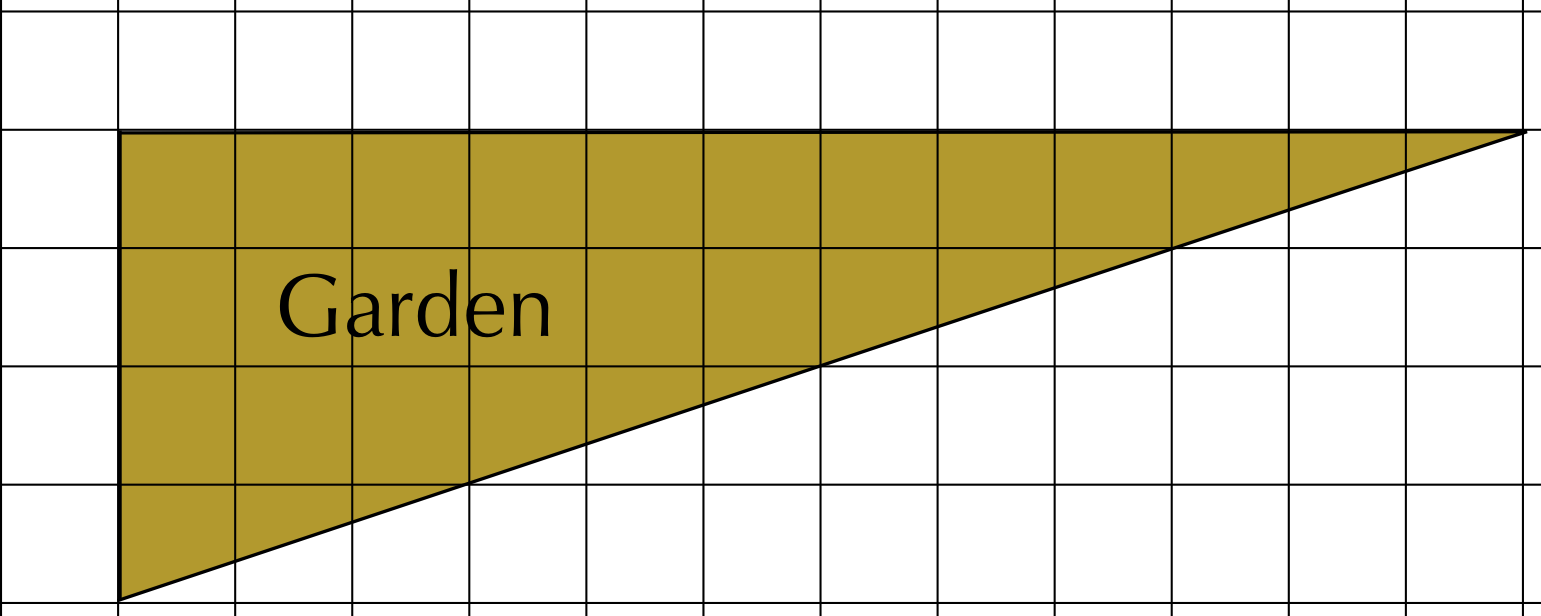• First, count the number of blocks whole blocks that fit inside the triangle. There are $$\text{18}$$ blocks that are completely inside the perimeter of the triangle.
• Next, count the number of half-blocks that fit inside the triangle, and divide the total by $$\text{2}$$. There are $$\text{4}$$ half-blocks covered by the triangle. This is equivalent to $$\text{2}$$ whole blocks.
• Now we count the remaining blocks and estimate how many whole blocks they would make up. There are $$\text{9}$$ blocks left that are approximately equivalent to $$\text{4}\frac{\text{1}}{\text{2}}$$ whole blocks.
• Add up the above numbers of blocks: $$\text{18}$$ + $$\text{2}$$ + $$\text{4,5}$$ = $$\text{24,5}$$ blocks. $$\text{24,5}$$ blocks = $$\text{24,5}$$ $$\text{cm}$$$$^{\text{2}}$$

## Worked example 6: Measuring and estimating the area of a circle using a grid

Using the grid below, estimate the area of the circular fish pond. Each square in the grid is $$\text{1}$$ $$\text{cm}$$ by $$\text{1}$$ $$\text{cm}$$.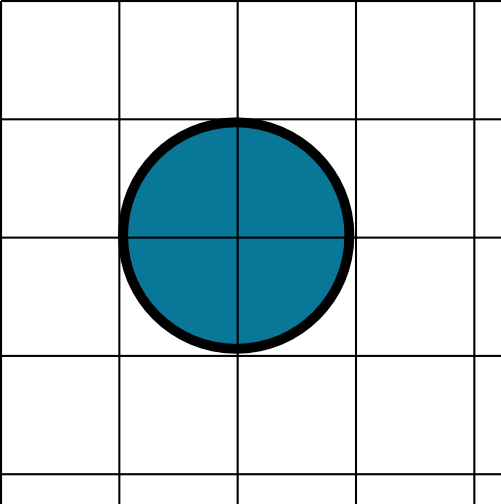As with the area of a triangle, we can only estimate the area of a circle using a grid. In the above diagram, Each piece of the circle takes up approximately three quarters of a square. There are four such squares, therefore we have:

$$\frac{\text{3}}{\text{4}} \times \text{4} = \frac{\text{12}}{\text{4}} = \text{3}$$ whole blocks $$\text{3}$$ whole blocks = $$\text{3}$$ $$\text{cm}$$$$^{\text{2}}$$

### Using formulae to calculate area (EMG5X)

We saw in the last two worked examples that estimating area using a grid is not always easy or very accurate. With the area of Mrs Dlamini's garden and the fish pond, we were at best guessing how many squares the outer edges of the shape covered. In order to accurately calculate the area of a shape, without estimating with a grid, there are simple formulae we can use:

 Shape Area formula Rectangle length $$\times$$ widthSquare length $$\times$$ length = length$$^{\text{2}}$$ and/or side $$\times$$ side = side$$^{\text{2}}$$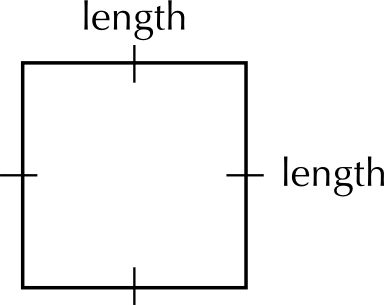and/or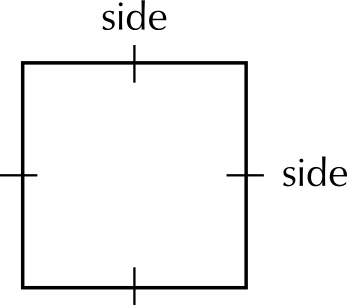Triangle $$\frac{\text{1}}{\text{2}} \times$$ base $$\times$$ perpendicular height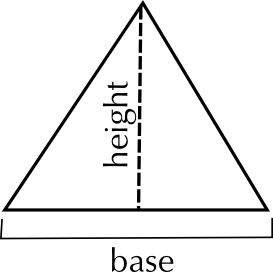or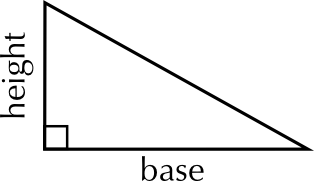Circle $$\pi \times$$ radius$$^{\text{2}}$$